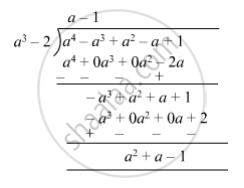SSC (English Medium) Class 8Maharashtra State Board
Share

# Divide and Write the Quotient and the Remainder. (A4 − A3 + A2 − a + 1) ÷ (A3 − 2) - SSC (English Medium) Class 8 - Mathematics

ConceptTo Divide a Polynomial by a Binomial

#### Question

Divide and write the quotient and the remainder.

(a4 − a3 + a2 − a + 1) ÷ (a3 − 2)

#### Solution

(a4 − a3 + a2 − a + 1) ÷ (a3 − 2)So, quotient = $a - 1$ and remainder = $a^2 + a - 1$

Is there an error in this question or solution?

#### APPEARS IN

Balbharati Solution for Balbharati Class 8 Mathematics (2019 to Current)
Chapter 10: Division of Polynomials
Practice Set 10.2 | Q: 6 | Page no. 66
Solution Divide and Write the Quotient and the Remainder. (A4 − A3 + A2 − a + 1) ÷ (A3 − 2) Concept: To Divide a Polynomial by a Binomial.
S